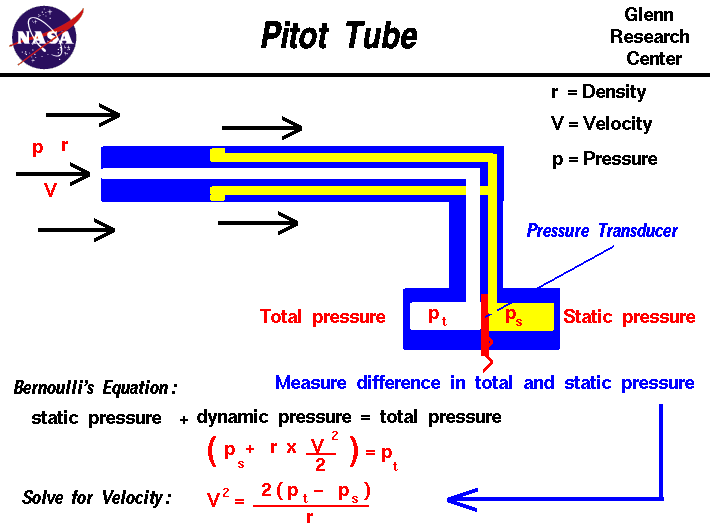+ Text Only Site
+ Non-Flash Version
+ Contact GlennThis page shows a schematic drawing of a pitot tube. Pitot tubes are used on aircraft as speedometers. The actual tube on the aircraft is around 10 inches (25 centimeters) long with a 1/2 inch (1 centimeter) diameter. Several small holes are drilled around the outside of the tube and a center hole is drilled down the axis of the tube. The outside holes are connected to one side of a device called a pressure transducer. The center hole in the tube is kept separate from the outside holes and is connected to the other side of the transducer. The transducer measures the difference in pressure in the two groups of tubes by measuring the strain in a thin element using an electronic strain gauge. The pitot tube is mounted on the aircraft so that the center tube is always pointed in the direction of travel and the outside holes are perpendicular to the center tube. On some airplanes the pitot tube is put on a longer boom sticking out of the nose of the plane or the wing. Difference in Static and Total Pressure Since the outside holes are perpendicular to the direction of travel, these tubes are pressurized by the local random component of the air velocity. The pressure in these tubes is the static pressure (ps) discussed in Bernoulli's equation. The center tube, however, is pointed in the direction of travel and is pressurized by both the random and the ordered air velocity. The pressure in this tube is the total pressure (pt) discussed in Bernoulli's equation. The pressure transducer measures the difference in total and static pressure which is the dynamic pressure q. measurement = q = pt - ps Solve for Velocity With the difference in pressures measured and knowing the local value of air density r from pressure and temperature measurements, we can use Bernoulli's equation to give us the velocity. Bernoulli's equation states that the static pressure plus one half the density times the velocity V squared is equal to the total pressure. ps + .5 * r * V ^2 = pt Solving for V: V ^2 = 2 * {pt - ps} / r V = sqrt [2 * {pt - ps} / r ] where sqrt denotes the square root function. There are, however, some practical limitations to this equation: If the velocity is low, the difference in pressures is very small and hard to accurately measure with the transducer. Errors in the instrument could be greater than the measurement! So pitot tubes don't work very well for very low velocities. If the velocity is very high (supersonic), we've violated the assumptions of Bernoulli's equation and the measurement is wrong again. At the front of the tube, a shock wave appears that will change the total pressure. There are corrections for the shock wave that can be applied to allow us to use pitot tubes for high speed aircraft. Activities:Guided ToursPitot Tube:Navigation ..Beginner's Guide Home Page+ Inspector General Hotline + Equal Employment Opportunity Data Posted Pursuant to the No Fear Act + Budgets, Strategic Plans and Accountability Reports + Freedom of Information Act + The President's Management Agenda + NASA Privacy Statement, Disclaimer, and Accessibility CertificationEditor: Tom Benson NASA Official: Tom Benson Last Updated: May 13 2021 + Contact Glenn